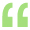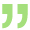# 87a rebate excel formula for ay 2020-21

If Total Income is less than equal to 500000 then Rebate under 87A will be 12500

Here is the Excel Formula.

Lets say Total Income Cell is A4 and tax cell is A5.

formula,   =if A4<=500000,MIN(A5,12500),0)

thankyou(Guest)
nice shubhamshubham singh (Job)     15 April 2019

w.e.f 1 April 2019, The Limit Is 500000Ankit Varshney   18 April 2019

 Originally posted by : rama krishnanit's not 500000 but it's 350000Satya N Dharani (Finance Professional)     22 April 2019

hi, shubam,

if(A4<=500000,MIN(A5,12500),0)

if a4=600000, a5=505000, formulae is not showing any difference, showing "0"

pl correct accoding its values.Satya N Dharani (Finance Professional)     22 April 2019

sorry , workingCA Vinod Singhvi (Partner at Rajesh Chheda and Company)     28 April 2020

For 87A REBATE CALCULATION KINDLY CHECK THIS FORMULA                                                                                                                =if (A4<=500000,A5,12500)

Thank youCA Vinod Singhvi (Partner at Rajesh Chheda and Company)     12 May 2020

FOR 87A REBATE THIS FORMULA IS MORE APPROPRIATE

=IF(A4<=500000,MIN(A5,12500),0)view more »